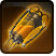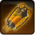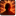CZ-M Synapse Accelerator SerumConditions

Related effects, buffs and debuffs

Please click on an effect below to view its details.

• [does not expire]CZ-M Synapse Accelerator Serum
In possession of one or more doses of CZ-M Synapse Accelerator Serum.

 Slot: Buff Duration: passive Tick rate: does not tick Initial stacks: 1 Max stacks: 100 # occurrences: 1 Tags: 5064585248198429032, 13497740320908092299
• On Apply

Only when the following conditions are met:

• If difficulty is set to Story Mode

Perform the following actions:

• Play appearance epp . operation . dxun . boss . holding_pens . player . grant_flare . buff, dependent on calling effect
• Unknown (167)
- Lock Out Player Abilities = (bool) false
- Temporary Package Spec = (int) 16141034770465696203
• Custom
- Param 2 = (string) Creating new story mode Stim owner
- Param 3 = (string)
- Param 4 = (string)
- Param 5 = (string)
- Param 6 = (string)
- Param 7 = (string)
- Param 8 = (string)
- Param 9 = (string)
- Param 1 = (int) 9499481598613081908
• On Apply

Only when the following conditions are met:

• If difficulty is set to Hard or Nightmare Mode

Perform the following actions:

• Play appearance epp . operation . dxun . boss . holding_pens . player . grant_flare . buff, dependent on calling effect
• Unknown (167)
- Lock Out Player Abilities = (bool) false
- Temporary Package Spec = (int) 16140946867632234890
• Custom
- Param 2 = (string) Creating new non-story mode Stim owner
- Param 3 = (string)
- Param 4 = (string)
- Param 5 = (string)
- Param 6 = (string)
- Param 7 = (string)
- Param 8 = (string)
- Param 9 = (string)
- Param 1 = (int) 9499481598613081908
• Just before NPC with this effect dies
• [target override]

Perform the following actions:

• Unknown (164)
- Ability Spec = (int) 16141013584623624930
- From Actor = (int) 2
- To Actor = (int) 2
• Custom
- Param 2 = (string) Flare Charge holder has died, Execute Drop Unlit Flares ability
- Param 3 = (string)
- Param 4 = (string)
- Param 5 = (string)
- Param 6 = (string)
- Param 7 = (string)
- Param 8 = (string)
- Param 9 = (string)
- Param 1 = (int) 9499481598613081908
• Just before this effect is removed

Perform the following actions:

• Dispel 99 effects from ability[stim_drop]

FQN: abl.​operation.​dxun.​boss.​holding_pens.​player.​stim_drop

[stim_drop]
• Custom
- Param 2 = (string) Flare Charge parent effect removed, cleaning up all associated children
- Param 3 = (string)
- Param 4 = (string)
- Param 5 = (string)
- Param 6 = (string)
- Param 7 = (string)
- Param 8 = (string)
- Param 9 = (string)
- Param 1 = (int) 9499481598613081908
• When TARGET gets revived

Perform the following actions:

• Dispel 99 effects
• Custom
- Param 2 = (string) We've been revived! Terminate stim granting AOE!
- Param 3 = (string)
- Param 4 = (string)
- Param 5 = (string)
- Param 6 = (string)
- Param 7 = (string)
- Param 8 = (string)
- Param 9 = (string)
- Param 1 = (int) 9499481598613081908
• When NPC with this effect receives an effect with tags 3482504472245890420
• [target override]

Only when the following conditions are met:

• <
• If stacks of this effect != 0
AND
• If TARGET is not alive
>

Perform the following actions:

• Custom
- Param 2 = (string) Valid Stim charge request received. Granting Stim charge.
- Param 3 = (string)
- Param 4 = (string)
- Param 5 = (string)
- Param 6 = (string)
- Param 7 = (string)
- Param 8 = (string)
- Param 9 = (string)
- Param 1 = (int) 9499481598613081908
• Add effect #2 to TARGET from TARGET
• When stacks of this effect decrease

Only when the following conditions are met:

• If stacks of this effect = 0

Perform the following actions:

• Custom
- Param 2 = (string) Zero stim charges. Removing effect.
- Param 3 = (string)
- Param 4 = (string)
- Param 5 = (string)
- Param 6 = (string)
- Param 7 = (string)
- Param 8 = (string)
- Param 9 = (string)
- Param 1 = (int) 9499481598613081908
• Remove this effect
• [0s] [not displayed]Effect #2

 Slot: Buff Duration: 0s Tick rate: does not tick # occurrences: 0 Tags: 5064585248198429032 Conditions: Can only by called by other effects
• On Apply
• [target override]

Perform the following actions:

• Modify Charges
- Ability Spec = (int) 0
- Amount Max = (float) -1
- Amount Min = (float) -1
- Amount Percent = (float) 0
• On Apply

Perform the following actions:

• Unknown (164)
- Ability Spec = (int) 16141102119333489615
- From Actor = (int) 3
- To Actor = (int) 3
• Custom
- Param 2 = (string) Stim request acknowledged. Executing Stim Grant.
- Param 3 = (string)
- Param 4 = (string)
- Param 5 = (string)
- Param 6 = (string)
- Param 7 = (string)
- Param 8 = (string)
- Param 9 = (string)
- Param 1 = (int) 9499481598613081908